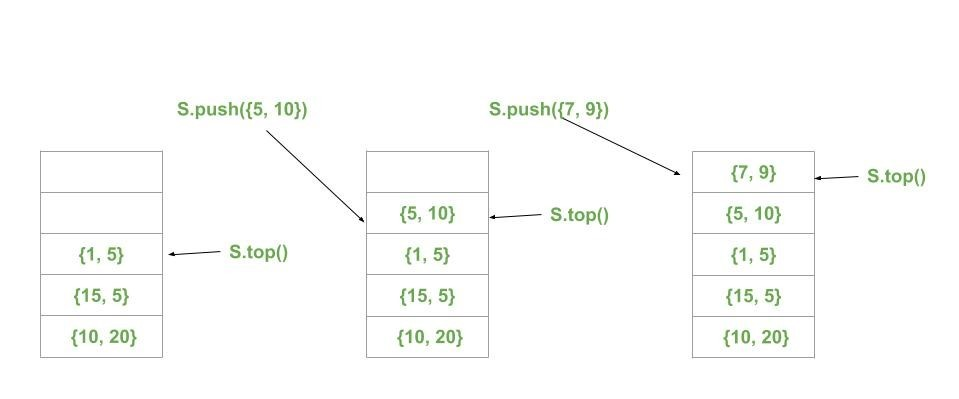# Stack of Pair in C++ STL with Examples

Stack in STL Stacks are a type of container adaptors with LIFO(Last In First Out) type of working, where a new element is added at one end and (top) an element is removed from that end only.

Pair in STL The pair container is a simple container defined in header consisting of two data elements or objects. The first element is referenced as ‘first’ and the second element as ‘second’ and the order is fixed (first, second).

Stack of pair in STL: Stack of pair can be very efficient in designing complex data structures.

Syntax:

```stack<pair<datatype, datatype>> stack_of_pair;
```

Below is an example to show the Stack of Pairs:

 `// CPP program to demonstrate ` `// the working of STL stack of pairs ` ` `  `#include ` `using` `namespace` `std; ` ` `  `// Print the current pair ` `void` `printPair(pair<``int``, ``int``> p) ` `{ ` ` `  `    ``cout << ``"("` `         ``<< p.first << ``", "` `         ``<< p.second << ``") "``; ` `} ` ` `  `// Print the Stack of Pairs ` `void` `Showstack(stack > s) ` `{ ` `    ``while` `(!s.empty()) { ` `        ``printPair(s.top()); ` `        ``s.pop(); ` `    ``} ` ` `  `    ``cout << ``'\n'``; ` `} ` ` `  `// Driver code ` `int` `main() ` `{ ` `    ``stack > s; ` ` `  `    ``s.push({ 10, 20 }); ` `    ``s.push({ 15, 5 }); ` `    ``s.push({ 1, 5 }); ` `    ``s.push({ 5, 10 }); ` `    ``s.push({ 7, 9 }); ` ` `  `    ``cout << ``"Stack of Pairs: "``; ` `    ``Showstack(s); ` ` `  `    ``cout << ``"\nSize of Stack of Pairs: "` `         ``<< s.size(); ` `    ``cout << ``"\nTop of Stack of Pairs: "``; ` `    ``printPair(s.top()); ` ` `  `    ``cout << ``"\n\nRemoving the top pair\n"``; ` `    ``s.pop(); ` ` `  `    ``cout << ``"Current Stack of Pairs: "``; ` `    ``Showstack(s); ` ` `  `    ``return` `0; ` `} `

Output:

```Stack of Pairs: (7, 9) (5, 10) (1, 5) (15, 5) (10, 20)

Size of Stack of Pairs: 5
Top of Stack of Pairs: (7, 9)

Removing the top pair
Current Stack of Pairs: (5, 10) (1, 5) (15, 5) (10, 20)
```

Below are the images to show the working of Stack of Pairs:Don’t stop now and take your learning to the next level. Learn all the important concepts of Data Structures and Algorithms with the help of the most trusted course: DSA Self Paced. Become industry ready at a student-friendly price.

My Personal Notes arrow_drop_upCheck out this Author's contributed articles.

If you like GeeksforGeeks and would like to contribute, you can also write an article using contribute.geeksforgeeks.org or mail your article to contribute@geeksforgeeks.org. See your article appearing on the GeeksforGeeks main page and help other Geeks.

Please Improve this article if you find anything incorrect by clicking on the "Improve Article" button below.